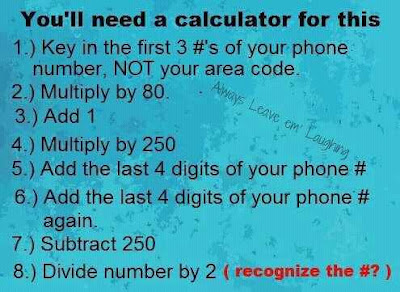## July 4, 2012

### Stupid Math Tricks

My friends on facebook have been passing around this stupid math trick:A lot of the math here is slight of hand. I'm working it out right now on the back of an envelope.

You can reduce the equation to (250(80a+1)+2b-250)/2=x

The "+1" is cancelled out after you multiply by 250, when you subtract 250 at the end (250*1=250). So you can just eliminate both of those.

Then you have 250(80a)+2b

Using 111's as your numbers and isolating the parts of the equation you can see the sort of effect that they have.

So if a=111 and b=1111 you can see the following:
250(80*111) = 2,220,000 Or twice the first three digits in your phone number.

When you "+2b", you just get twice the last four digits in your phone number.

When you then divide all those numbers in half, you get your phone number. It's a roundabout way of just entering your phone number into a calculator and then being surprised that you got your phone number.

You're welcome.